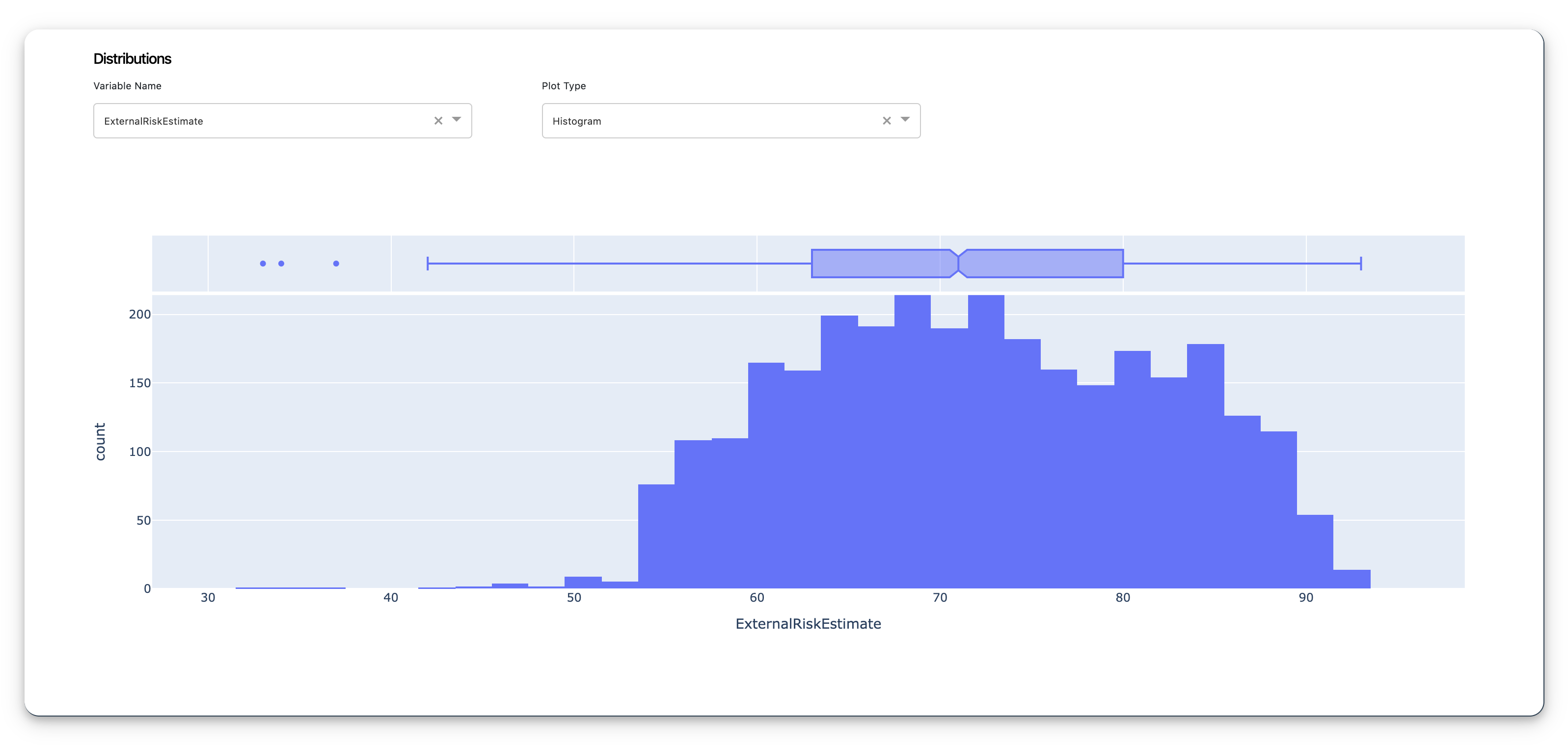# explainX.aiWelcome!

explainX.ai is an end to end explainable AI framework for model developers to fully understand, explain & debug their machine learning models.

### Analyzing theexplainX.ai Dashboard

#### Get a snapshot of your test data.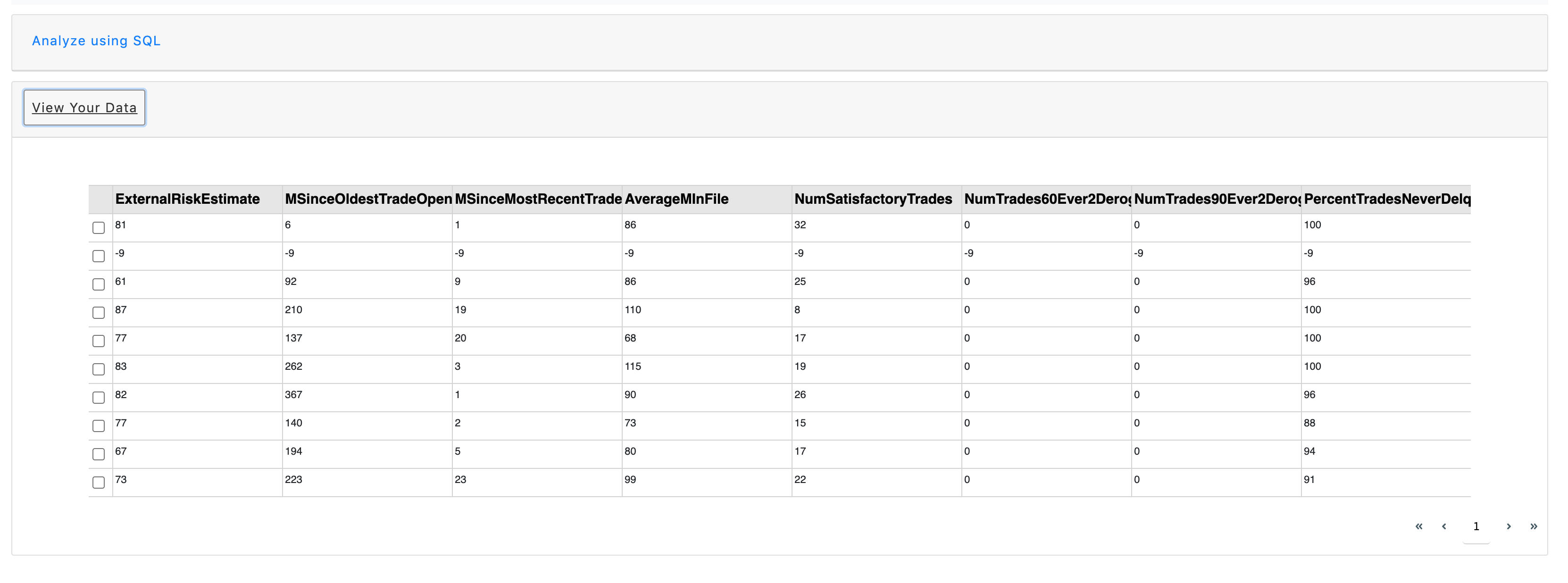## Global Feature Impact

#### The global feature impact identifies whether the variable had an overall positive impact or an overall negative impact, according to the model, on the overall prediction.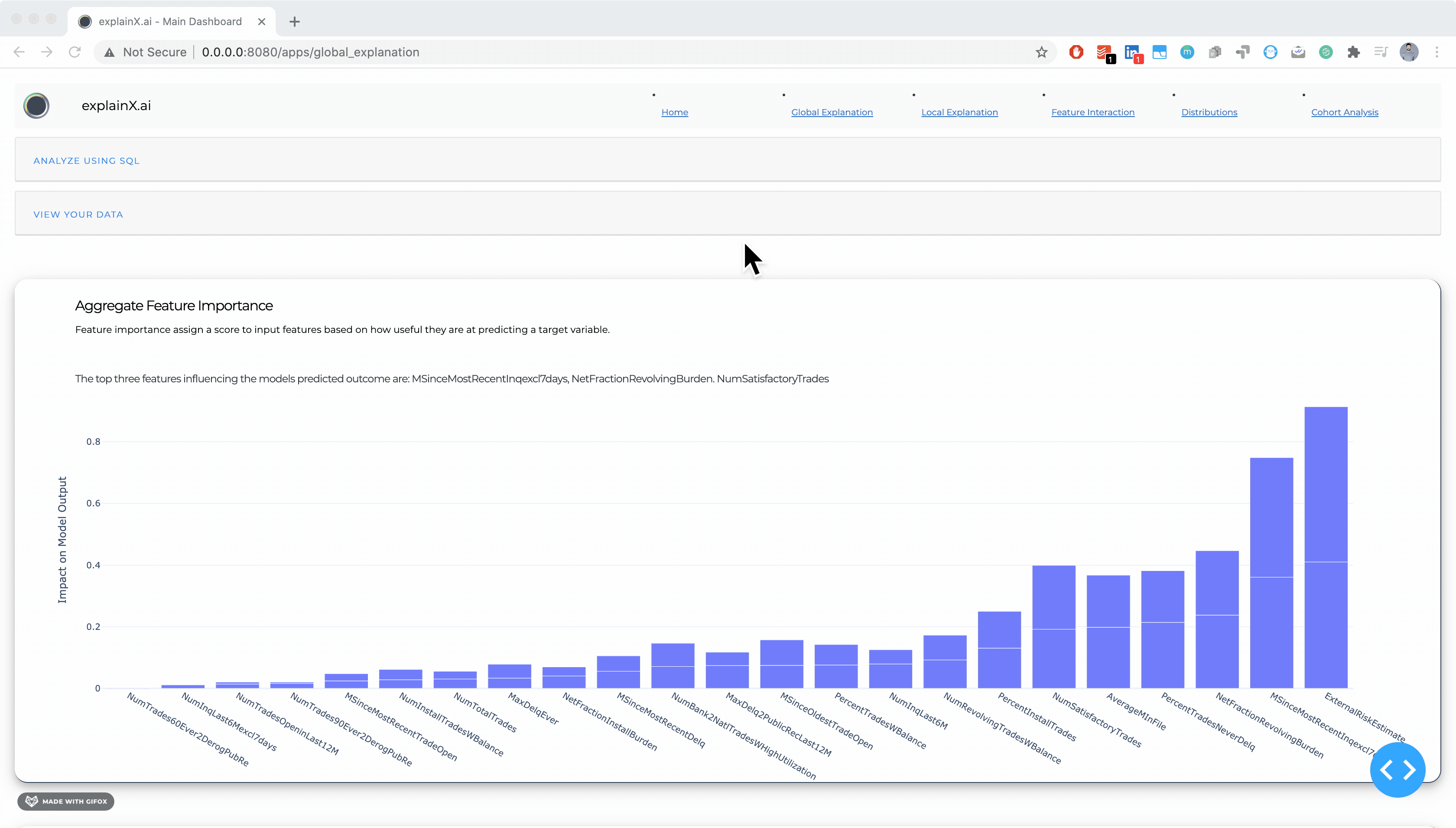## 3. Regional Level Explanation by using SQL

#### We can figure out how our model behaves in different subsets of our data. For example, the model might behave differently on customers in NYC than it did on customers in LA. So by using SQL query, adding these rules and exploring behavior on subset of data becomes extremely valuable.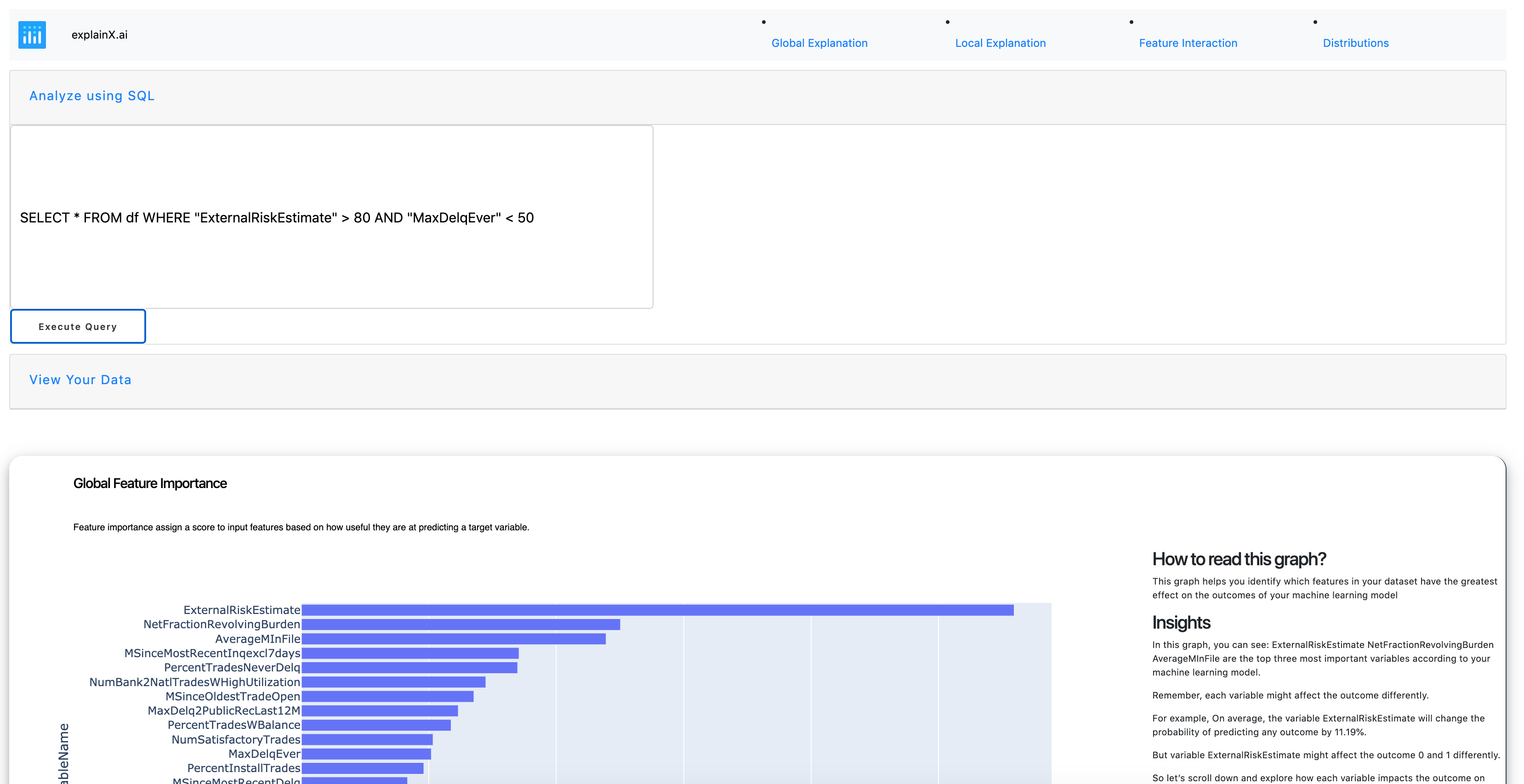## 4. Local Level Explanation for a Single Prediction Point

#### Next, you can zoom in on a single instance and examine what the model predicts for this input, and explain why. For each prediction point i.e. a new customer, the model might behave differently and it is important to identify that.One of the cool things about explainX is that our local level explanation is coupled with a what-if analysis form. The purpose of this form is to enable data scientists and business users simulate various scenarios and explore how the model behaves.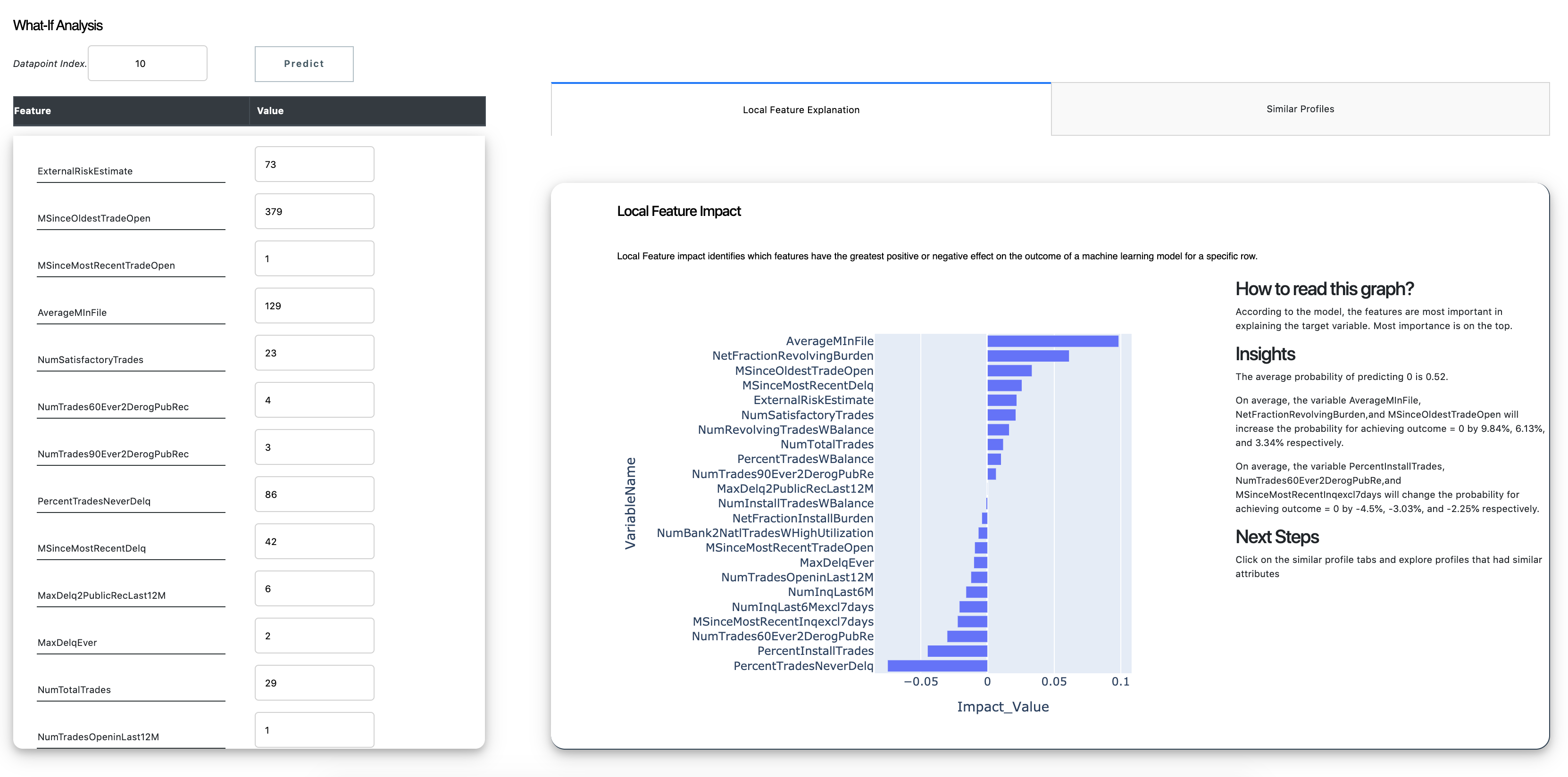## Similar Prototypes

#### We also understand that humans think through similar examples so in order to further dig deeper into how the model prediction, users can generate similar profiles from within the dataset that had similar attributes. These similar profiles show similarities in model prediction and our ground truth values.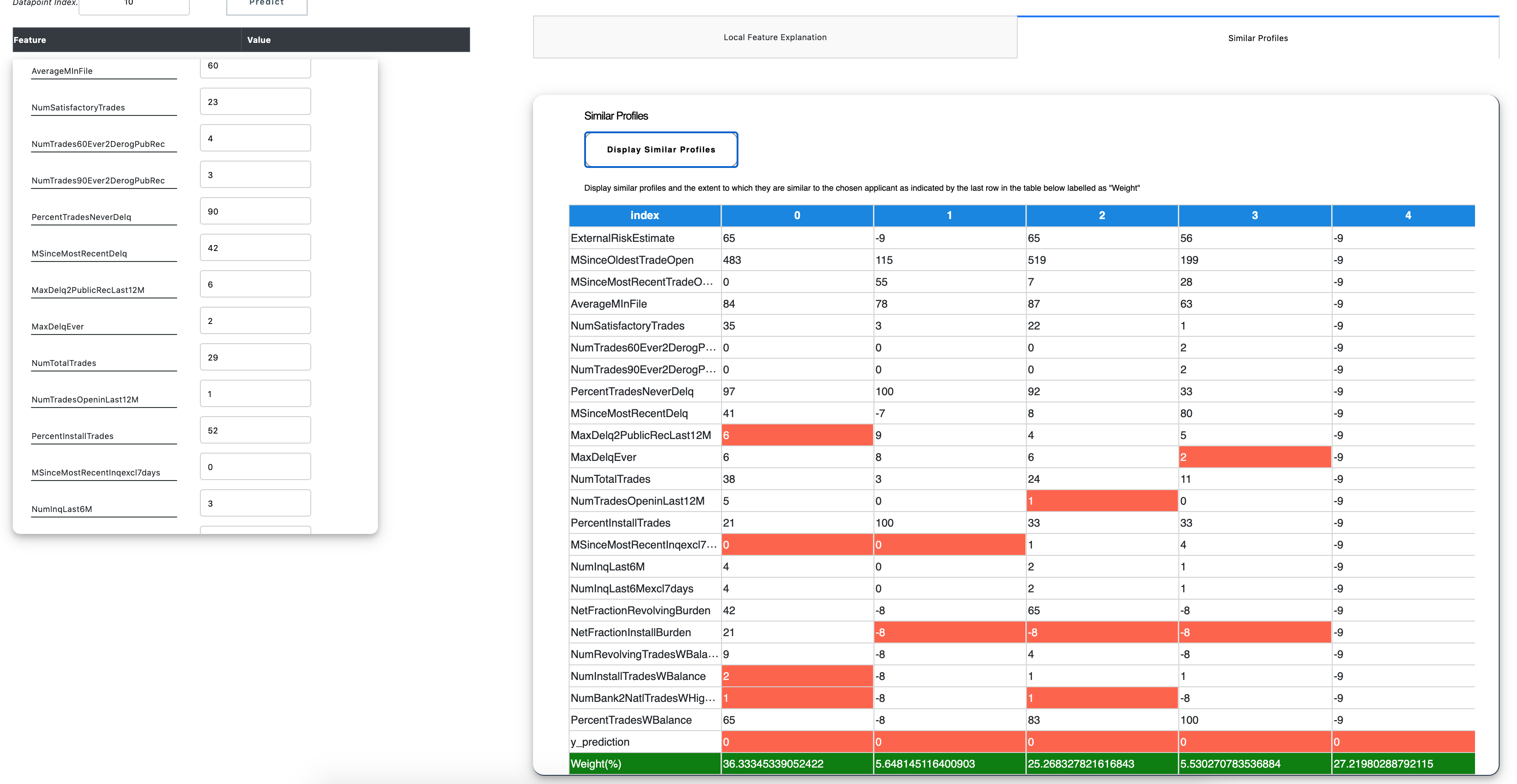## Partial Dependence Plot (PDP) Plot

#### The partial dependence plot (short PDP or PD plot) shows the marginal effect one or two features have on the predicted outcome of a machine learning model. In this example, we can see that as AGE increase, its impact on the predicting outcome also increase which results in a higher prediction (mean value of the house) represented by the increase color contrast.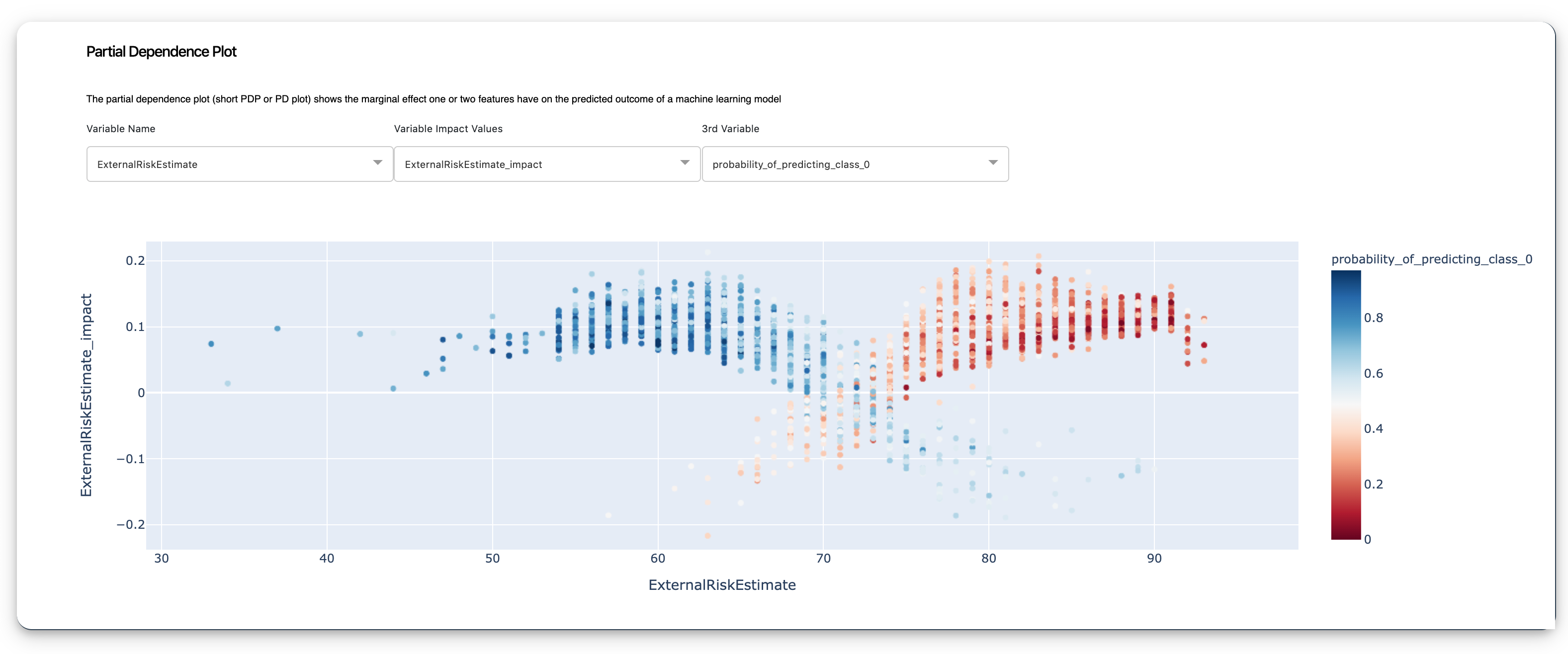## Summar Plot

#### The summary plot combines feature importance with feature effects. Each point on the summary plot is an impact value for a feature and an instance. The position on the y-axis is determined by the feature and on the x-axis by the impact value. The colour represents the value of the feature from low to high.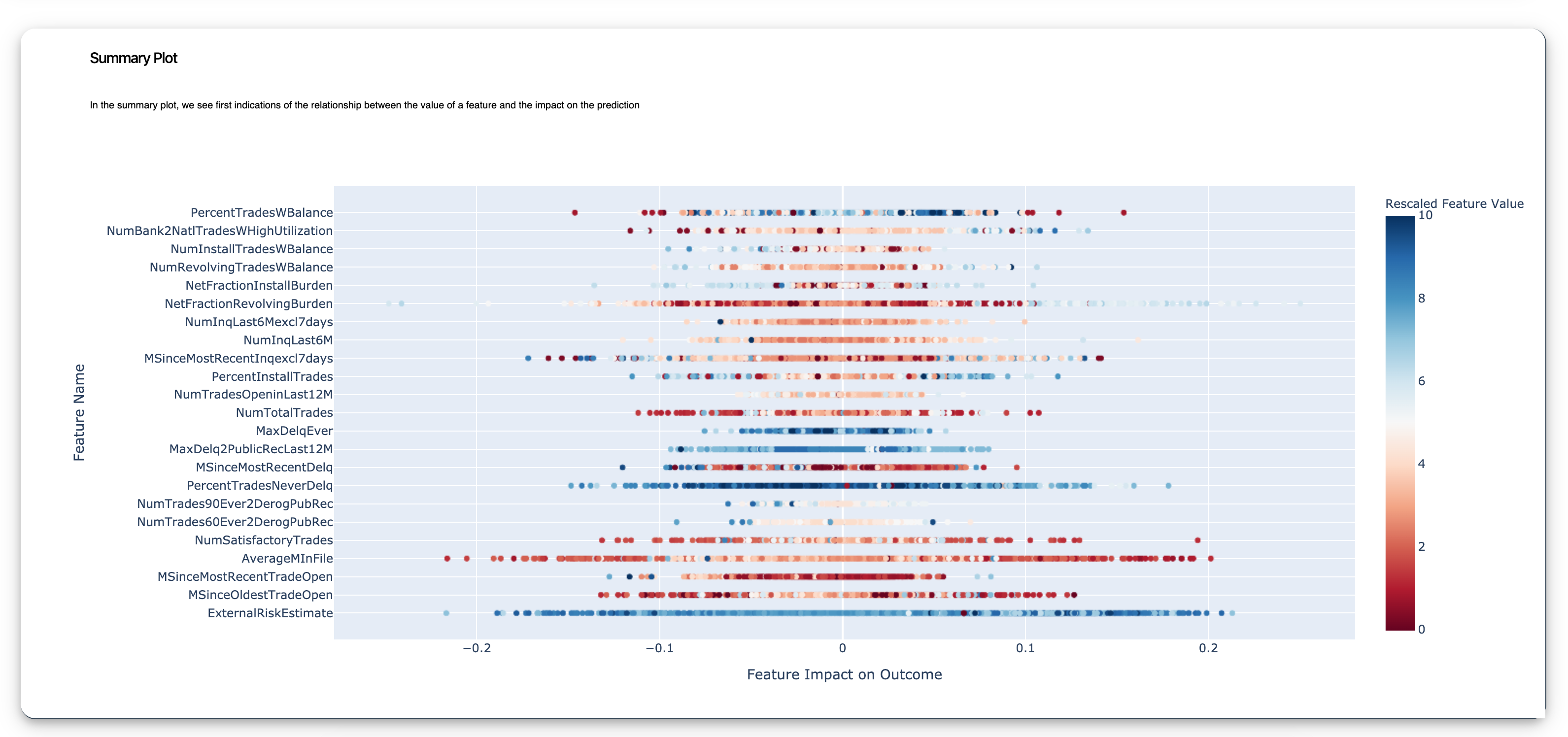## Histograms & Violet Plots

#### Use the histogram to get a distribution of your numerical or categorical variables. Use joint violet plots to get basic statistics summary like mean, median, model, Q1, Q3, Q4 for your variables. Now you can identify the distribution of the predicting variable on top of your other variables to find a join distribution.